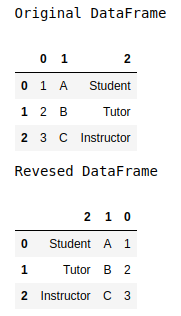Skip to content
Related Articles
How to reverse the column order of the Pandas DataFrame?
• Last Updated : 01 Jun, 2021

Sometimes when working with DataFrames we might want to change or reverse the order of the column of the dataframe. In this article, let’s see how to reverse the order of the columns of a dataframe. This can be achieved in two ways –

Method 1: The sequence of columns appearing in the dataframe can be reversed by using the attribute.columns[::-1] on the corresponding dataframe. It accesses the columns from the end and outer dataframe[…] reindexes the dataframe using this new sequence provided.

Example:

## Python3

 `# importing required modules``import` `pandas as pd`  `dataframe ``=` `pd.DataFrame([[``1``, ``'A'``, ``"Student"``],``                          ``[``2``, ``'B'``, ``"Tutor"``],``                          ``[``3``, ``'C'``, ``"Instructor"``]])` `print``(``"Original DataFrame"``)``display(dataframe)` `# reversing the dataframe``print``(``"Reversed DataFrame"``)``display(dataframe[dataframe.columns[::``-``1``]])`

Output:Method 2: iloc indexer can also be used to reverse the column order of the data frame, using the syntax iloc[:, ::-1] on the specified dataframe. The contents are not preserved in the original dataframe.

## Python3

 `# importing required modules``import` `pandas as pd`  `dataframe ``=` `pd.DataFrame([[``1``, ``'A'``, ``"Student"``],``                          ``[``2``, ``'B'``, ``"Tutor"``],``                          ``[``3``, ``'C'``, ``"Instructor"``]])` `print``(``"Original DataFrame"``)``display(dataframe)` `# reversing the dataframe``print``(``"Reversed DataFrame"``)``display(dataframe.iloc[:, ::``-``1``])`

Output:Attention geek! Strengthen your foundations with the Python Programming Foundation Course and learn the basics.

To begin with, your interview preparations Enhance your Data Structures concepts with the Python DS Course. And to begin with your Machine Learning Journey, join the Machine Learning – Basic Level Course

My Personal Notes arrow_drop_up